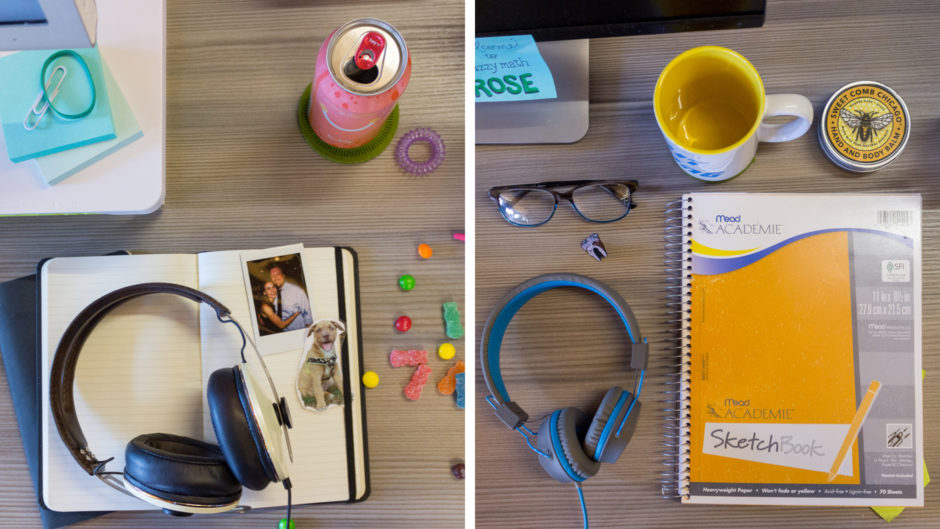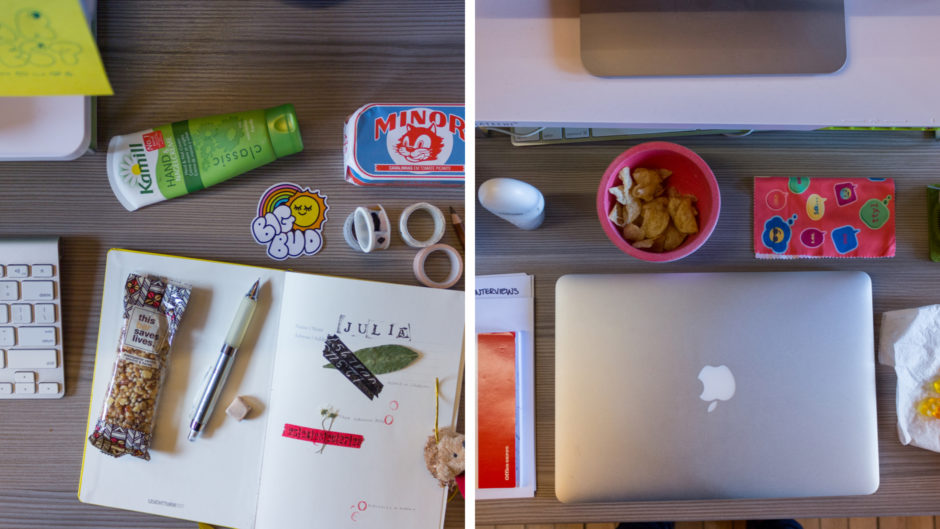Fuzzy Math Spotlight: Rachel V. & Nick

Posted by Fuzzy MathFuzzy Math Spotlight: Rachel S. & Rose

Posted by Fuzzy MathFuzzy Math Spotlight: Mia & Philip

Posted by Fuzzy MathFuzzy Math Spotlight: Kelly & Carl

Posted by Fuzzy MathFuzzy Math Spotlight: Liz & Paris

Posted by Fuzzy MathFuzzy Math Spotlight: Wren & Jon

Posted by Fuzzy MathFuzzy Math Spotlight: Julia & Rebecca

Posted by Fuzzy Math
We have a UX newsletter! Sign up for it or else.